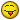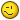# Circle at ending/starting points of polylines, based on number of intersecting polylines

## Recommended PostsHi, does anyone have a lisp which I can use for this:

I'm working on a piping network made of polylines. I need to insert circles at the ending/starting points of all polylines (without having multiple circles at the same location).

- The circle has to be blue if: 2 polylines have their start/end overlapping, or if the end/start of a polyline doesn't intersect with any other.

- But when 3 (or more) polylines share the same ending/starting point, the circle should be red.

Example drawing attached.

##### Share on other sitesPosted (edited)
```(defun c:test ( / 2col 2rad 3col 3rad lst n pt tol)
(setq 2rad 5	; <--- radius of circle when two intersecting points are found
2col acBlue	; <--- ACI color of circle when two intersecting points are found
3rad 5	; <--- radius of circle when three intersecting points are found
3col acRed	; <--- ACI color of circle when three intersecting points are found
tol 1e-7	; <--- the tolerance between intersecting points to be considered equal
)
(if
(setq lst
(apply 'append
(mapcar
'(lambda (x) (list (vlax-curve-getStartPoint x) (vlax-curve-getEndPoint x)))
(JH:selset-to-list (ssget '((0 . "LWPOLYLINE"))))
)
)
)
(while lst
(setq pt (car lst)
n (<=
(-
(length lst)
(length (setq lst (vl-remove-if '(lambda (y) (equal y pt tol)) lst)))
)
2
)
)
(entmake
(list
'(0 . "CIRCLE")
'(100 . "AcDbCircle")
(cons 10 pt)
(cons 62 (if n 2col 3col))
)
)
)
)
(princ)
)

;; JH:selset-to-list --> Jonathan Handojo
;; Returns a list of entities from a selection set
;; ss - selection set

(defun JH:selset-to-list (selset / lst iter)
(if selset
(repeat (setq iter (sslength selset))
(setq lst (cons (ssname selset (setq iter (1- iter))) lst))
)
)
)```

Edited by Jonathan Handojo
•1

##### Share on other sitesHello, something from me```;; Group by Number  -  Lee Mac
;; Groups a list 'l' into a list of lists, each of length 'n'

(defun LM:group-n ( l n / r )
(if l
(cons
(reverse (repeat n (setq r (cons (car l) r) l (cdr l)) r))
(LM:group-n l n)
)
)
)

(entmakex (list (cons 0 "CIRCLE")
(cons 10 cen)
(cons 62 clr))))

;Create from Georgi Georgiev - TRUDY
;Date 07.01.2021

(defun c:try1 ( / allpoint2 end1)
(setq allcord '())
(setq sscord (ssget (list (cons 0 "LWPOLYLINE"))))
(repeat (setq i (sslength sscord))
(setq Vname (vlax-ename->vla-object (ssname sscord (setq i (1- i)))))
(setq tcord (vlax-get Vname 'coordinates))
(setq allcord (append tcord allcord))
(setq allcord2 (LM:group-n allcord 2))

(setq startL (car allcord2))
(setq endL (car (reverse allcord2)))
(setq allcord2 nil allcord nil)
(setq allpoint2 (append (append startL endL) allpoint2))
)
(setq allpoint3 (LM:group-n allpoint2 2))
(setq allpoint4 allpoint3)
;;;;;;;;;;; remove multiple
(while allpoint3
(setq remM (cons (car allpoint3) remM))
(setq allpoint3 (vl-remove (car allpoint3) allpoint3))
)
(setq end2 '())
(repeat (length remM)
(setq remM2 (car remM))
(setq allpoint5 allpoint4)
(setq n 0)
(repeat (length allpoint5)
(if (equal remM2 (car allpoint5) 0.00001) (progn (setq n (+ n 1)) (setq end1 (cons n end1))))
(setq allpoint5 (cdr allpoint5))

)
(if end1 (setq end2 (cons (append remM2 (list (length end1))) end2)))
(setq end1 nil)
(setq remM (cdr remM))
)

(foreach x end2 (if (< 2 (nth 2 x)) (circle (list (nth 0 x) (nth 1 x)) rad 1) (circle (list (nth 0 x) (nth 1 x)) rad 5)))
(princ)
)
```

•1

##### Share on other sitesThis should be more than enough to get the job done I believe.```(defun c:Test ( / int sel ent get pts crd fnd )
;; Tharwat - 07.Jan.2021	;;
(and (princ "\nSelect polylines : ")
(setq int -1 sel (ssget '((0 . "LWPOLYLINE"))))
(while (setq int (1+ int) ent (ssname sel int))
(setq get (entget ent)
pts (cons (cdr (assoc 10 get)) pts)
pts (cons (cdr (assoc 10 (reverse get))) pts)
)
)
(while (setq crd (car pts))
(setq pts (cdr pts) fnd nil)
(foreach itm pts
(and (equal crd itm 1e-4)
(setq fnd (cons itm fnd)
pts (vl-remove itm pts)
)
)
)
(setq fnd (cons crd fnd))
(entmake (list '(0 . "CIRCLE") (cons 10 crd) '(40 . 5.0) (cons 62 (if (<= (length fnd) 2) 5 1))))
)
)
(princ)
)```

•1

##### Share on other sites3 hours ago, Trudy said:

Hello, something from me```
;; Group by Number  -  Lee Mac
;; Groups a list 'l' into a list of lists, each of length 'n'

(defun LM:group-n ( l n / r )
(if l
(cons
(reverse (repeat n (setq r (cons (car l) r) l (cdr l)) r))
(LM:group-n l n)
)
)
)

(entmakex (list (cons 0 "CIRCLE")
(cons 10 cen)
(cons 62 clr))))

;Create from Georgi Georgiev - TRUDY
;Date 07.01.2021

(defun c:try1 ( / allpoint2 end1)
(setq allcord '())
(setq sscord (ssget (list (cons 0 "LWPOLYLINE"))))
(repeat (setq i (sslength sscord))
(setq Vname (vlax-ename->vla-object (ssname sscord (setq i (1- i)))))
(setq tcord (vlax-get Vname 'coordinates))
(setq allcord (append tcord allcord))
(setq allcord2 (LM:group-n allcord 2))

(setq startL (car allcord2))
(setq endL (car (reverse allcord2)))
(setq allcord2 nil allcord nil)
(setq allpoint2 (append (append startL endL) allpoint2))
)
(setq allpoint3 (LM:group-n allpoint2 2))
(setq allpoint4 allpoint3)
;;;;;;;;;;; remove multiple
(while allpoint3
(setq remM (cons (car allpoint3) remM))
(setq allpoint3 (vl-remove (car allpoint3) allpoint3))
)
(setq end2 '())
(repeat (length remM)
(setq remM2 (car remM))
(setq allpoint5 allpoint4)
(setq n 0)
(repeat (length allpoint5)
(if (equal remM2 (car allpoint5) 0.00001) (progn (setq n (+ n 1)) (setq end1 (cons n end1))))
(setq allpoint5 (cdr allpoint5))

)
(if end1 (setq end2 (cons (append remM2 (list (length end1))) end2)))
(setq end1 nil)
(setq remM (cdr remM))
)

(foreach x end2 (if (< 2 (nth 2 x)) (circle (list (nth 0 x) (nth 1 x)) rad 1) (circle (list (nth 0 x) (nth 1 x)) rad 5)))
(princ)
)
```

Ooh, Trudy, you should localize your variables within the 'try1' function. You do know what happens when you don't, right? If I end up writing other functions using those variables, their values may carry over to my function (should I not localize them) and cause my function to malfunction or not work as expected.

`( / allcord allcord2 allpoint2 allpoint3 allpoint4 allpoint5 end1 end2 endl i n rad remm remm2 sscord startl tcord vname)`

##### Share on other sitesPosted (edited)
51 minutes ago, Tharwat said:

This should be more than enough to get the job done I believe.```
(defun c:Test ( / int sel ent get pts crd fnd )
;; Tharwat - 07.Jan.2021	;;
(and (princ "\nSelect polylines : ")
(setq int -1 sel (ssget '((0 . "LWPOLYLINE"))))
(while (setq int (1+ int) ent (ssname sel int))
(setq get (entget ent)
pts (cons (cdr (assoc 10 get)) pts)
pts (cons (cdr (assoc 10 (reverse get))) pts)
)
)
(while (setq crd (car pts))
(setq pts (cdr pts) fnd nil)
(foreach itm pts
(and (equal crd itm 1e-4)
(setq fnd (cons itm fnd)
pts (vl-remove itm pts)
)
)
)
(setq fnd (cons crd fnd))
(entmake (list '(0 . "CIRCLE") (cons 10 crd) '(40 . 5.0) (cons 62 (if (<= (length fnd) 2) 5 1))))
)
)
(princ)
)```

Wow... nice and short. Same idea to my approach, just that I had a function to convert stuff, so I got lazy to just loop through selection sets manually.

Though sometimes I wonder... does vl-remove work on points? That's why I opt to use vl-remove-if

Edited by Jonathan Handojo
Removed modified code

##### Share on other sites17 minutes ago, Jonathan Handojo said:

Here's a shorter one. I'll leave it to you to spot the differenceReally ?

Excuse me, I don't like anyone to modify my codes for any reason to come up with any idea to show off.

So just write yours or learn from the other 's posted codes.

##### Share on other sites1 minute ago, Tharwat said:

Really ?

Excuse me, I don't like anyone to modify my codes for any reason to come up with any idea to show off.

So just write yours or learn from the other 's posted codes.

Sorry, my apologies. I didn't expect it to leave a negative reaction. I've removed it.

##### Share on other sitesPosted (edited)
On 1/7/2021 at 10:35 PM, Jonathan Handojo said:

Sorry, my apologies. I didn't expect it to leave a negative reaction. I've removed it.

No worries, thanks for understanding.

Edited by Tharwat
typo

##### Share on other sites```(defun C:test ( / sL SS pL cda cL )

(setq sL '((fuzz . 1e-2) (rad . 5) (cEl . 5) (cTY . 1))); settings

(princ "\nSelect polylines: ")
(if (setq SS (ssget "_:L-I" '((0 . "LWPOLYLINE"))))
(setq
pL (mapcar ''((e) (apply 'append (mapcar ''((x) (if (= 10 (car x)) (list (cdr x)))) (entget e)))) (vl-remove-if 'listp (mapcar 'cadr (ssnamex SS))))
sL
(append sL
'( (_cir (p c r) (entmakex (list '(0 . "CIRCLE") (cons 10 p) (cons 40 r) (cons 62 c))))
(cdrassoc (k L) (cdr (assoc k l)))
)
)
cda (cdr (assoc 'cdrassoc sL))
SS
(foreach p (apply 'append pL)
(cond
( (vl-some ''((cp) (and (equal (cdr cp) p (cda 'fuzz sL)) (setq cL (subst (cons (1+ (car cp)) (cdr cp)) cp cL)))) cL) )
( (setq cL (cons (cons 0 p) cL)) )
); cond
); foreach
SS
(foreach p cL
(
'((a b)
(cond
( (< 0 a 2) ((cda '_cir sL) b (cda 'cEl sL) (cda 'rad sL)) )
( (>= a 2)  ((cda '_cir sL) b (cda 'cTY sL) (cda 'rad sL)) )
( (and (= 0 a ) (vl-some ''( (ep) (equal ep b (cda 'fuzz sL))) (append (mapcar 'last pL) (mapcar 'car pL))))
( (cda '_cir sL) b (cda 'cEl sL) (cda 'rad sL) )
)
)
)
(car p) (cdr p)
)
); foreach
SS '(just playing around)
); setq
); and
(princ)
); defun ```

•1

##### Share on other sitesAll work flawlessly, except Tharwat's and Jonathan's are much faster to execute. so I'll be using those.

Thanks everyone, you're all very helpful!##### Share on other sitesPosted (edited)

@enthralled Another to try .. should be quite a bit faster on large selection sets.

```(defun c:foo (/ a n s x)
;; RJP » 2021-01-08
(cond	((setq s (ssget '((0 . "LWPOLYLINE"))))
(setq s (vl-remove-if 'listp (mapcar 'cadr (ssnamex s))))
(setq s (mapcar '(lambda (x) (list (vlax-curve-getstartpoint x) (vlax-curve-getendpoint x))) s))
(setq s (vl-sort (apply 'append s) '(lambda (r j) (< (car r) (car j)))))
(setq s (vl-sort s '(lambda (r j) (< (cadr r) (cadr j)))))
(while	(car s)
(setq a (car s))
(setq s (cdr s))
(setq n 0)
(while (equal a (car s) 1e-8) (setq n (1+ n)) (setq s (cdr s)))
(entmake (list '(0 . "CIRCLE") (cons 10 a) '(8 . "Circle") '(40 . 5) (cons 62 (if (> n 1) 1 5))))
)
)
)
(princ)
)```

A wheeeee bit faster on 100x the OP sample drawingQuote

<Selection set: 52> Benchmarking .....Elapsed milliseconds / relative speed for 4 iteration(s):

(FOO SS)...............2672 / 60.69 <fastest>
(JHTEST SS)...........31890 / 5.08
(THARWATTEST SS).....162156 / 1.00 <slowest>

_\$

Edited by ronjonp
•1

## Join the conversation

You can post now and register later. If you have an account, sign in now to post with your account.
Note: Your post will require moderator approval before it will be visible.×   Pasted as rich text.   Paste as plain text instead

Only 75 emoji are allowed.